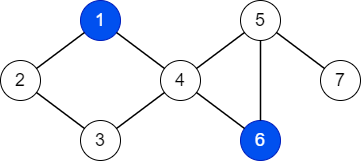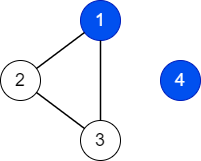## Blue graph

View as PDF

Points: 0.80 (partial)
Time limit: 2.0s
Memory limit: 256M
Input: stdin
Output: stdout

Problem type
Allowed languages
C, C++, Java, Kotlin, Pascal, PyPy, Python, Scratch

You are given an undirected graph with ~n~ vertices and ~m~ edges, in which no edges connect a node to itself, as well as no pair of nodes are connected by more than one edge. Initially, each node in the graph is colored white. Your task is to color some nodes blue, such that ~q~ requirements are satisfied, the ~i~-th requirement stating that the shortest distance from node ~c_i~ to any blue node (possibly itself) is exactly ~d_i~.

#### Input

The first line contains three integers ~n~, ~m~ and ~q~ (~1 \le q \le n \le 5 \times 10^5~, ~0 \le m \le 5 \times 10^5~), denoting the number of nodes, the number of edges, and the number of requirements, respectively.

Each of the next ~m~ lines contains two integers ~u~ and ~v~ (~1 \le u, v \le n~, ~u \ne v~), denoting an edge between the two nodes ~u~ and ~v~. It is guaranteed that no pair of nodes are connected by more than one edge.

The next ~q~ lines, the ~i~-th of which contains two integers ~c_i~ and ~d_i~ (~1 \le c_i \le n~, ~0 \le d_i < n~) denoting the ~i~-th condition: the shortest distance from node ~c_i~ to any blue node (possibly itself) is exactly ~d_i~. It is guaranteed that each node appears in at most one condition.

#### Output

If there does not exist a coloring satisfying all requirements, output NO.

Otherwise, output YES on the first line. On the second line, output a single integer ~k~, the number of nodes to be colored blue. The third line, output ~k~ integers ~p_1, p_2, \ldots, p_k~ (~p_i \ne p_j~ for ~i \ne j~), the indices of the nodes to be colored blue.

If there are multiple valid colorings, you may output any.

#### Scoring

Not the final scoring

• Subtask 1 (~10~ points): ~n \le 16~.

• Subtask 2 (~20~ points): ~n \le 5000~.

The total score for this problem is ~100~.

#### Sample Input 1

7 8 3
1 2
2 3
3 4
4 1
4 5
5 6
6 4
5 7
7 2
4 1
2 1


#### Sample Output 1

YES
2
1 6


#### Sample Input 2

4 3 1
1 2
2 3
3 1
4 0


#### Sample Output 2

YES
2
1 4


#### Sample Input 3

3 3 1
1 2
2 3
3 1
1 2


#### Sample Output 3

NO


#### Notes

In the first example, we can color two nodes ~1~ and ~6~ blue:

• From node ~7~, the closest blue node is node ~6~ can be reached by following the path ~7 \rightarrow 5 \rightarrow 6~.

• From node ~4~, the closest blue node is node ~1~ can be reached by following the path ~4 \rightarrow 1~.

• From node ~2~, the closest blue node is node ~1~ can be reached by following the path ~2 \rightarrow 1~.Illustration of the first sample.

Another solution would be to color the nodes ~3~ and ~6~ blue.

In the second example, we can color the node ~4~ blue. The other nodes can be colored arbitrarily.Illustration of the second sample.

In the third example, the distance from node ~1~ to both of the other two nodes is ~1~, therefore it is impossible to color a node such that the closest distance from ~1~ to a blue node is exactly ~2~.